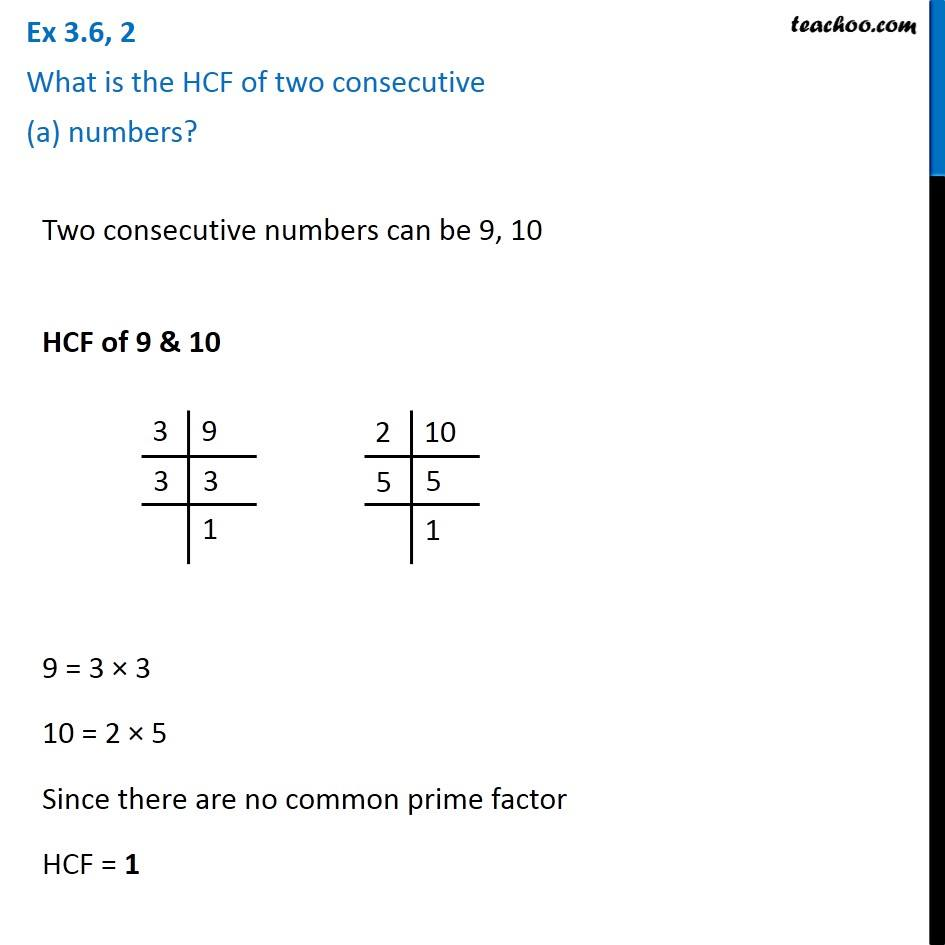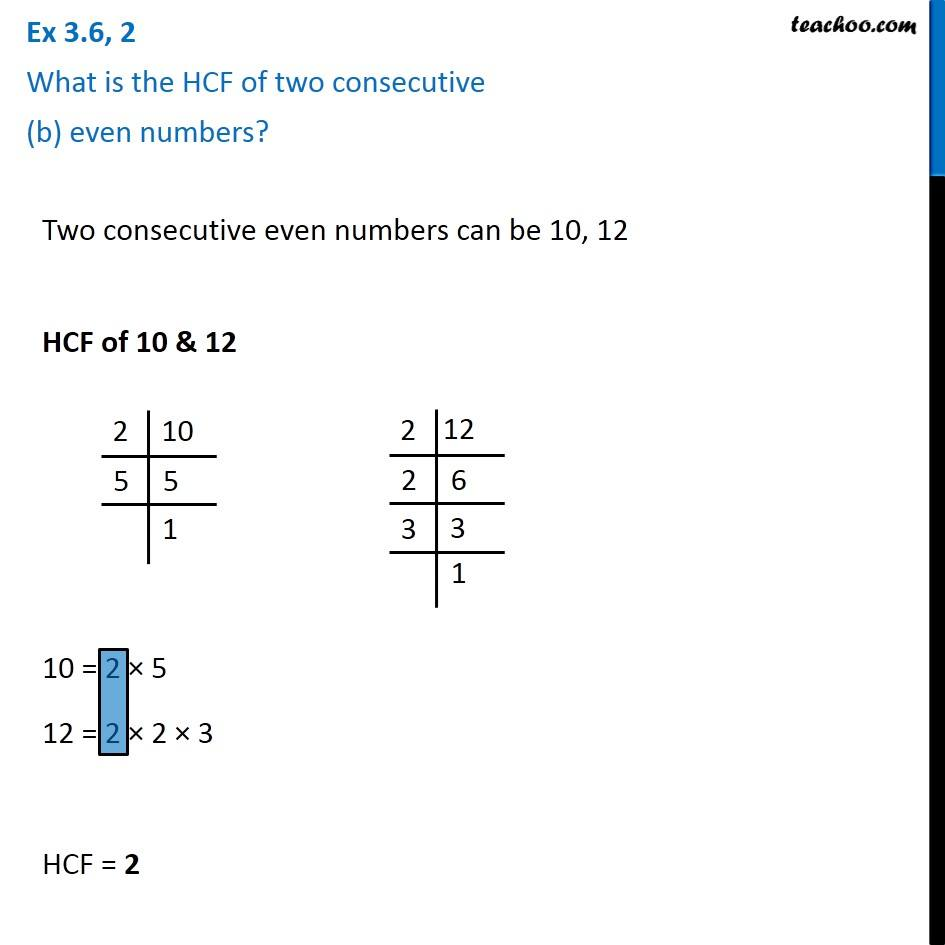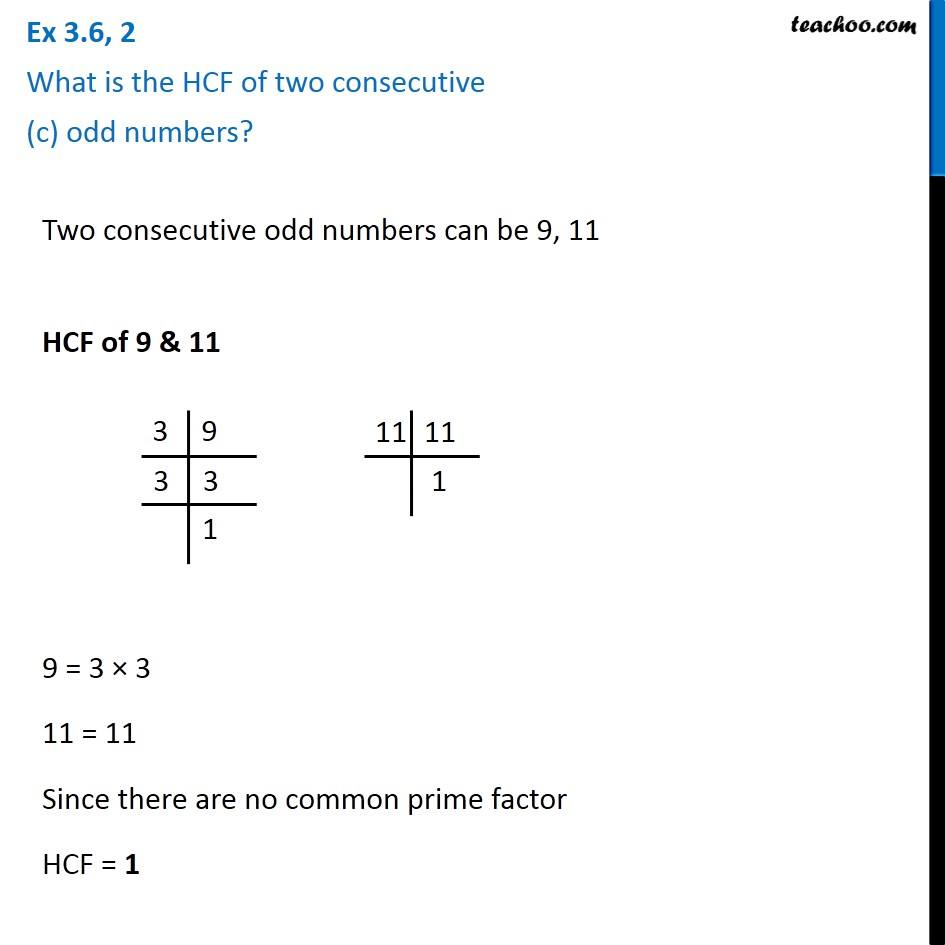Ex 3.6

Chapter 3 Class 6 Playing with Numbers
Serial order wiseLearn in your speed, with individual attention - Teachoo Maths 1-on-1 Class

### Transcript

Ex 3.6, 2 What is the HCF of two consecutive (a) numbers? Two consecutive numbers can be 9, 10 HCF of 9 & 10 3|9 3|3 |1 2|10 5|5 |1 9 = 3 × 3 10 = 2 × 5 Since there are no common factor HCF = 1 Ex 3.6, 2 What is the HCF of two consecutive (b) even numbers? Two consecutive even numbers can be 10, 12 2|10 5|5 |1 2|12 2|6 3|2 |1 HCF of 10 & 12 Ex 3.6, 2 What is the HCF of two consecutive (c) odd numbers? Two consecutive odd numbers can be 9, 11 HCF of 9 & 11 3|9 3|3 |1 2|11 2|1 9 = 3 × 3 11 = 11 Since there are no common factor HCF = 1